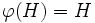# Quotient-coprime automorphism-faithful subgroup

## Contents

BEWARE! This term is nonstandard and is being used locally within the wiki. [SHOW MORE]
This article defines a subgroup property: a property that can be evaluated to true/false given a group and a subgroup thereof, invariant under subgroup equivalence. View a complete list of subgroup properties[SHOW MORE]

## Definition

A subgroup$H$ of a finite group$G$ is termed quotient-coprime automorphism-faithful if$H$ is a normal subgroup of$G$, and:

If$\varphi$ is a non-identity automorphism of$G$ whose order is relatively prime to the order of$G$, such that$\varphi(H) = H$, then the induced map by$\varphi$ on$G/H$ is also a non-identity automorphism.